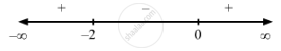Share

F(X) = (X − 1) (X+2)2. - CBSE (Arts) Class 12 - Mathematics

Question

f(x) =  (x $-$ 1) (x+2)2

Solution

$\text { Given }: f\left( x \right) = \left( x - 1 \right) \left( x + 2 \right)^2$

$\Rightarrow f'\left( x \right) = \left( x + 2 \right)^2 + 2\left( x + 2 \right)\left( x - 1 \right)$

$\text{ For a local maximum or a local minimum, we must have }$

$f'\left( x \right) = 0$

$\Rightarrow \left( x + 2 \right)^2 + 2\left( x + 2 \right)\left( x - 1 \right) = 0$

$\Rightarrow \left( x + 2 \right)\left( x + 2 + 2x - 2 \right) = 0$

$\Rightarrow \left( x + 2 \right)\left( 3x \right) = 0$

$\Rightarrow x = 0, - 2$Since  f '(x) changes from negative to positive when x increases through 0, x = 0 is the point of local minima.

The local minimum value of  f (x) at x = 0 is given by $\left( 0 - 1 \right) \left( 0 + 2 \right)^2 = - 4$

Since  f '(x) changes sign from positive to negative when x increases through $- 2$ ,x = $- 2$ is the point of local maxima.
The local maximum value of  f (x)  at x = $- 2$ is given by

$\left( - 2 - 1 \right) \left( - 2 + 2 \right)^2 = 0$
Is there an error in this question or solution?

Video TutorialsVIEW ALL 

Solution F(X) = (X − 1) (X+2)2. Concept: Graph of Maxima and Minima.
S